# Functions CS 202 Epp section Aaron Bloomfield 1

• Slides: 30
Download presentation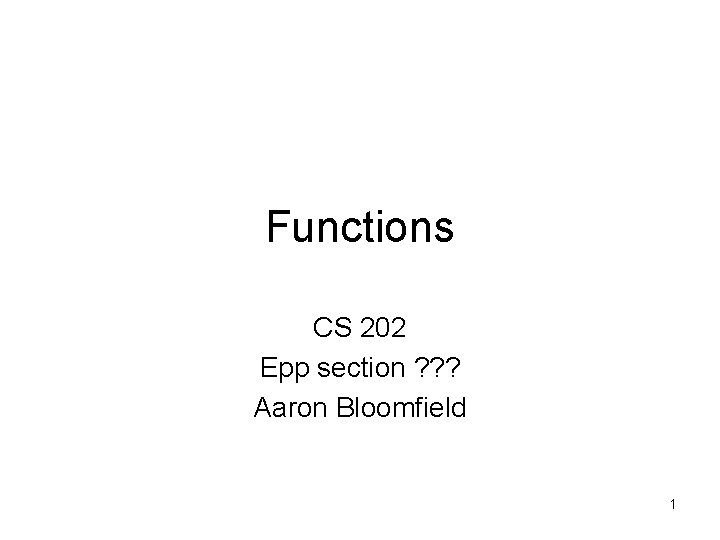Functions CS 202 Epp section ? ? ? Aaron Bloomfield 1Definition of a function • A function takes an element from a set and maps it to a UNIQUE element in another set 2Function terminology f maps R to Z Domain R f Z Co-domain f(4. 3) 4 4. 3 Pre-image of 4 Image of 4. 3 3More functions A pre-image of 1 The image of A Domain Co-domain Alice A “a” 1 Bob B “bb“ 2 Chris C “cccc” 3 Dave D “dd” 4 Emma F “e” 5 A class grade function A string length function 4Even more functions Range a 1 “a” 1 e 2 “bb“ 2 i 3 “cccc” 3 o 4 “dd” 4 u 5 “e” 5 Some function… Not a valid function! Also not a valid function! 5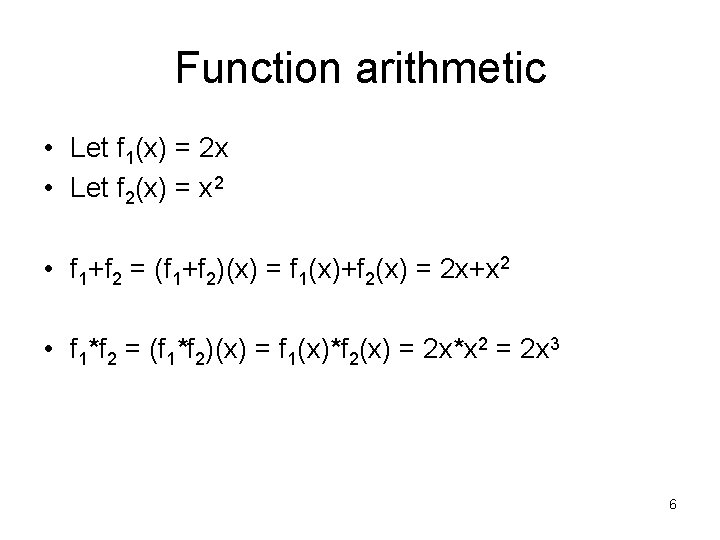Function arithmetic • Let f 1(x) = 2 x • Let f 2(x) = x 2 • f 1+f 2 = (f 1+f 2)(x) = f 1(x)+f 2(x) = 2 x+x 2 • f 1*f 2 = (f 1*f 2)(x) = f 1(x)*f 2(x) = 2 x*x 2 = 2 x 3 6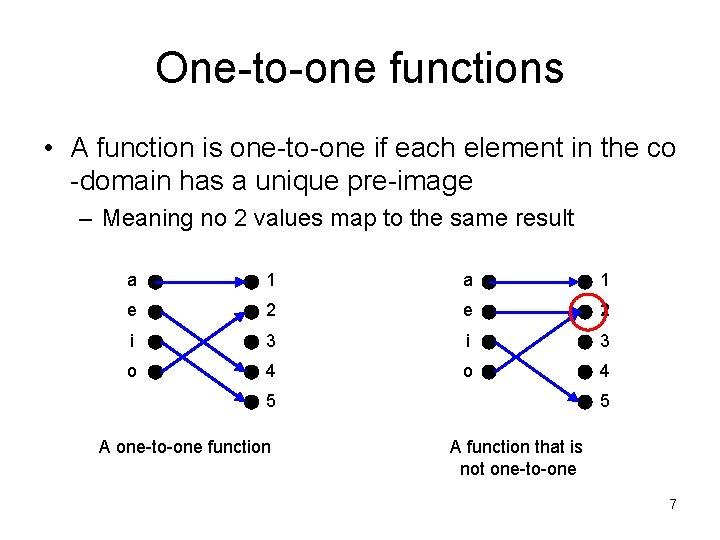One-to-one functions • A function is one-to-one if each element in the co -domain has a unique pre-image – Meaning no 2 values map to the same result a 1 e 2 i 3 o 4 5 A one-to-one function 5 A function that is not one-to-one 7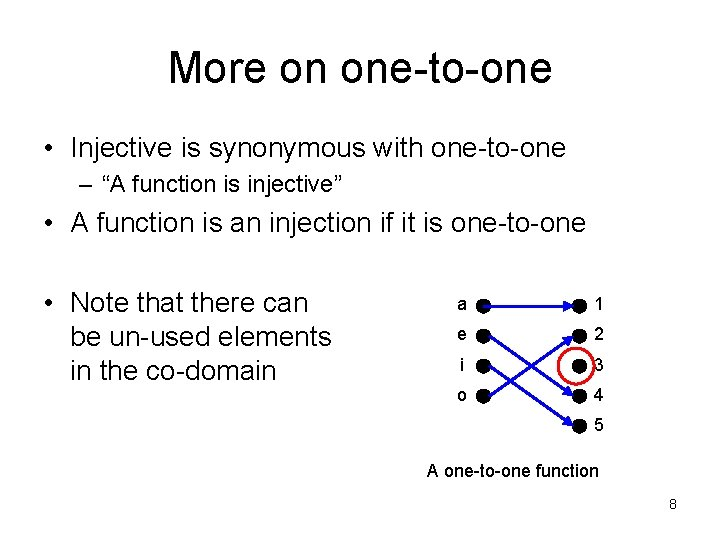More on one-to-one • Injective is synonymous with one-to-one – “A function is injective” • A function is an injection if it is one-to-one • Note that there can be un-used elements in the co-domain a 1 e 2 i 3 o 4 5 A one-to-one function 8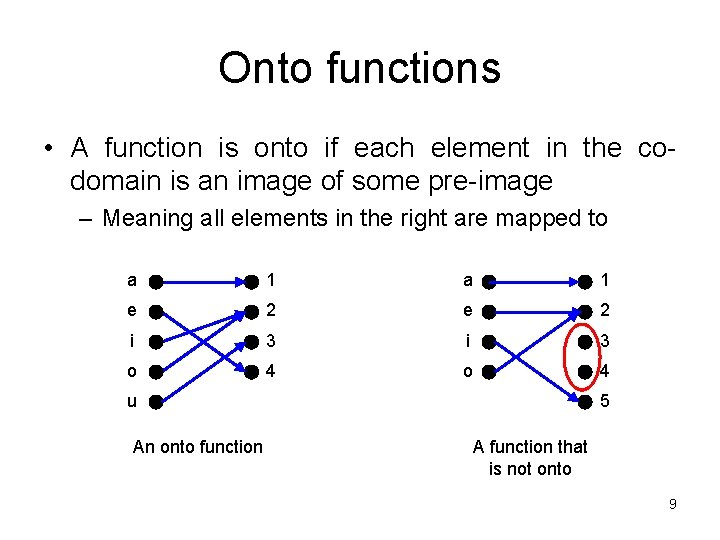Onto functions • A function is onto if each element in the codomain is an image of some pre-image – Meaning all elements in the right are mapped to a 1 e 2 i 3 o 4 u An onto function 5 A function that is not onto 9More on onto • Surjective is synonymous with onto – “A function is surjective” • A function is an surjection if it is onto • Note that there can be multiply used elements in the co-domain a 1 e 2 i 3 o 4 u An onto function 10Onto vs. one-to-one • Are the following functions onto, one-to-one, both, or neither? a 1 b 2 c 3 4 d 4 1 -to-1, not onto Both 1 -to-1 and onto a 1 b 2 c 3 d 4 d Onto, not 1 -to-1 Neither 1 -to-1 nor onto a 1 b 2 c 3 4 Not a valid function 11Bijections • Consider a function that is both one-to-one and onto: a 1 b 2 c 3 d 4 • Such a function is a one-to-one correspondence, or a bijection 12Identity functions • A function such that the image and the preimage are ALWAYS equal • f(x) = 1*x • f(x) = x + 0 • The domain and the co-domain must be the same set 13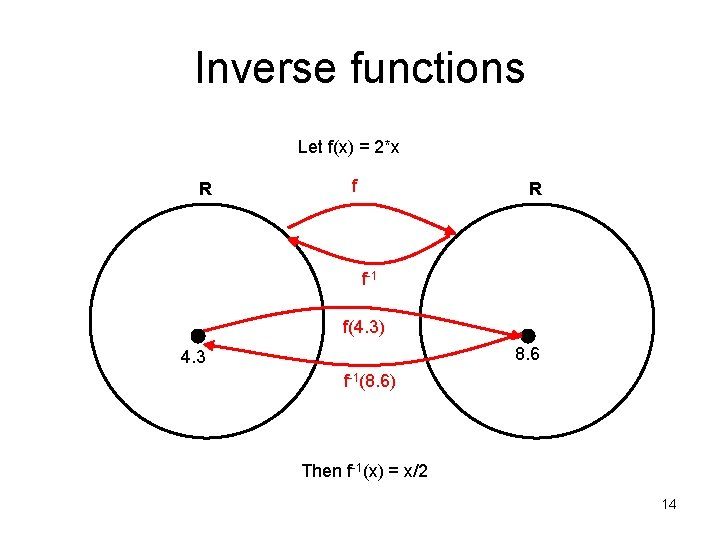Inverse functions Let f(x) = 2*x R f-1 f(4. 3) 8. 6 4. 3 f-1(8. 6) Then f-1(x) = x/2 14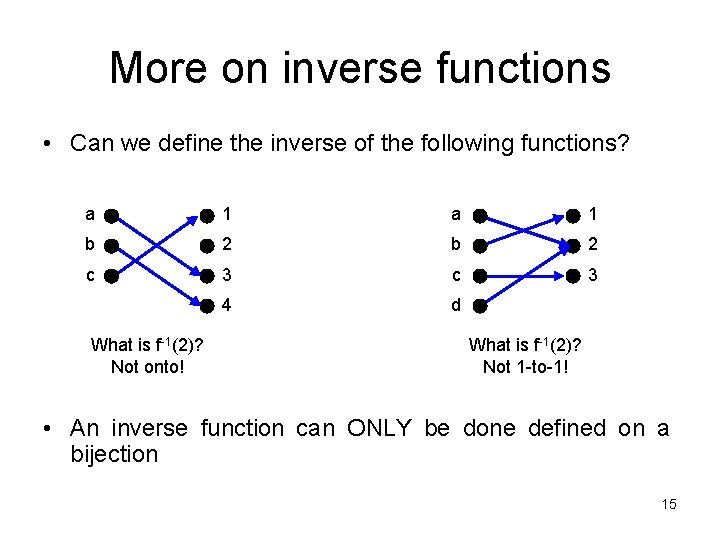More on inverse functions • Can we define the inverse of the following functions? a 1 b 2 c 3 4 d What is f-1(2)? Not onto! What is f-1(2)? Not 1 -to-1! • An inverse function can ONLY be done defined on a bijection 15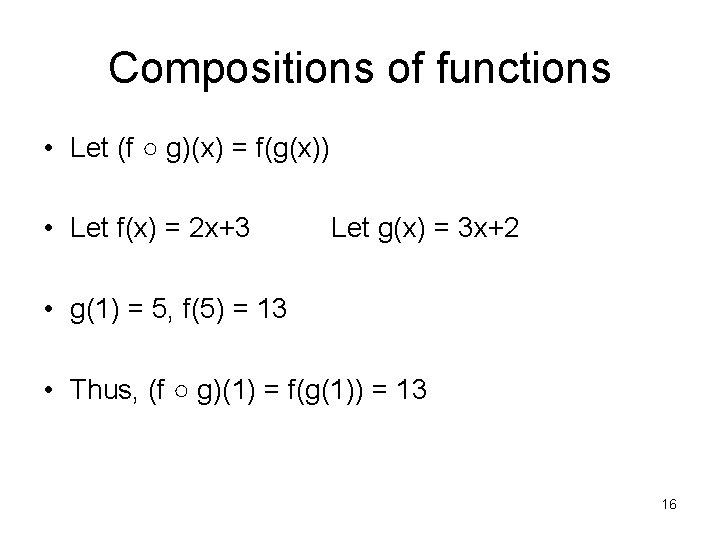Compositions of functions • Let (f ○ g)(x) = f(g(x)) • Let f(x) = 2 x+3 Let g(x) = 3 x+2 • g(1) = 5, f(5) = 13 • Thus, (f ○ g)(1) = f(g(1)) = 13 16Compositions of functions f○g A B C g f g(a) f(a) a g(a) f(g(a)) (f ○ g)(a) 17Compositions of functions Let f(x) = 2 x+3 f○g R Let g(x) = 3 x+2 R R g f g(1) f(5) f(g(1))=13 1 g(1)=5 (f ○ g)(1) f(g(x)) = 2(3 x+2)+3 = 6 x+7 18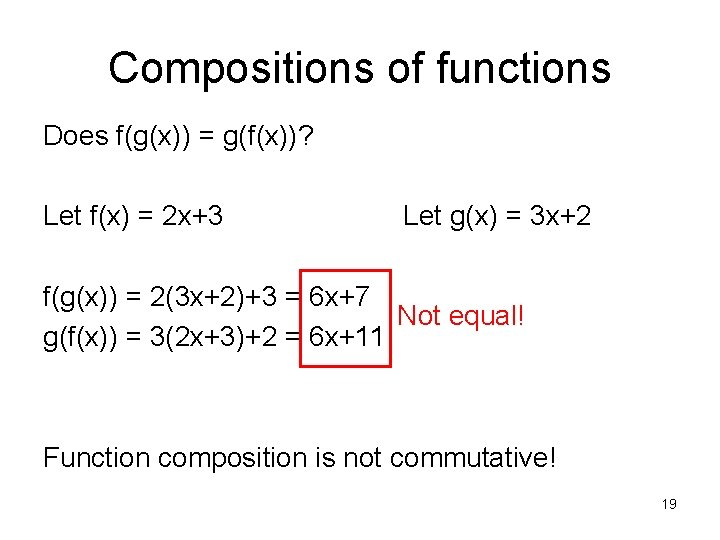Compositions of functions Does f(g(x)) = g(f(x))? Let f(x) = 2 x+3 Let g(x) = 3 x+2 f(g(x)) = 2(3 x+2)+3 = 6 x+7 Not equal! g(f(x)) = 3(2 x+3)+2 = 6 x+11 Function composition is not commutative! 19Graphs of functions f(x)=3 x=1 Let f(x)=2 x+1 Plot (x, f(x)) f(x)=5 x=2 This is a plot of f(x) 20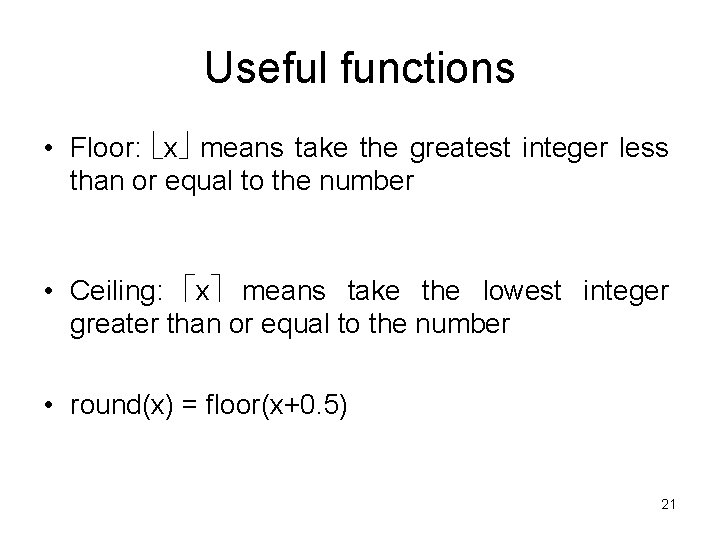Useful functions • Floor: x means take the greatest integer less than or equal to the number • Ceiling: x means take the lowest integer greater than or equal to the number • round(x) = floor(x+0. 5) 21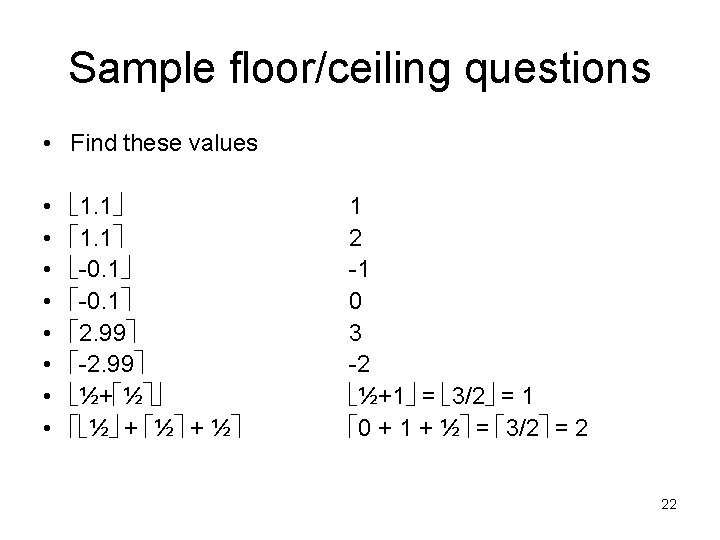Sample floor/ceiling questions • Find these values • • 1. 1 -0. 1 2. 99 -2. 99 ½+ ½ ½ + ½ 1 2 -1 0 3 -2 ½+1 = 3/2 = 1 0 + 1 + ½ = 3/2 = 2 22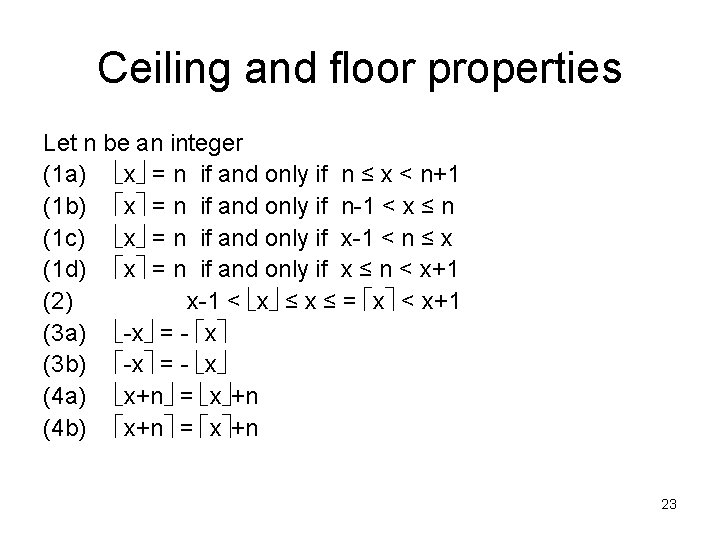Ceiling and floor properties Let n be an integer (1 a) x = n if and only if n ≤ x < n+1 (1 b) x = n if and only if n-1 < x ≤ n (1 c) x = n if and only if x-1 < n ≤ x (1 d) x = n if and only if x ≤ n < x+1 (2) x-1 < x ≤ = x < x+1 (3 a) -x = - x (3 b) -x = - x (4 a) x+n = x +n (4 b) x+n = x +n 23Ceiling property proof • Prove rule 4 a: x+n = x +n – Where n is an integer – Will use rule 1 a: x = n if and only if n ≤ x < n+1 • Direct proof! – – – Let m = x Thus, m ≤ x < m+1 (by rule 1 a) Add n to both sides: m+n ≤ x+n < m+n+1 By rule 4 a, m+n = x+n Since m = x , m+n also equals x +n Thus, x +n = m+n = x+n 24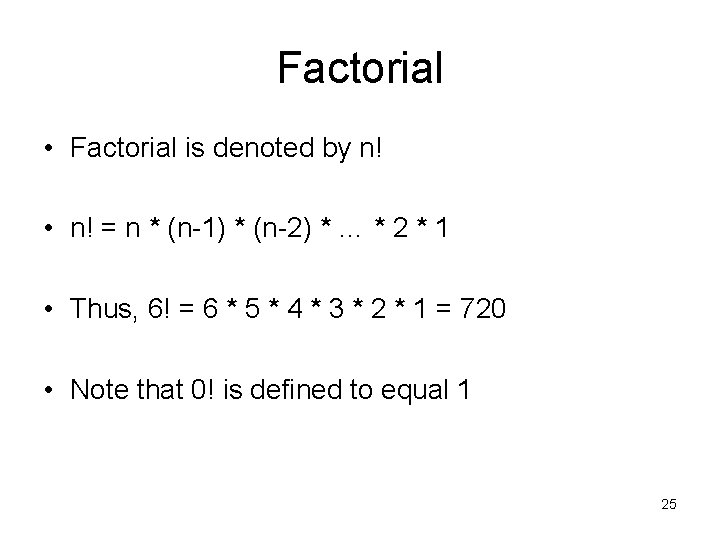Factorial • Factorial is denoted by n! • n! = n * (n-1) * (n-2) * … * 2 * 1 • Thus, 6! = 6 * 5 * 4 * 3 * 2 * 1 = 720 • Note that 0! is defined to equal 1 25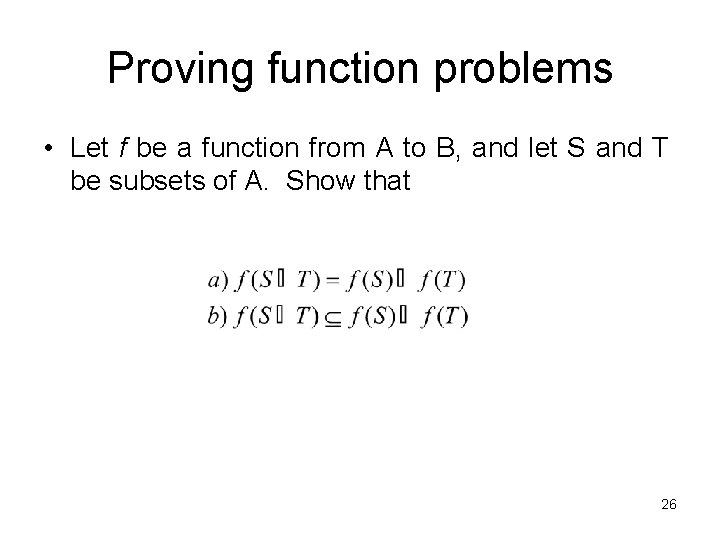Proving function problems • Let f be a function from A to B, and let S and T be subsets of A. Show that 26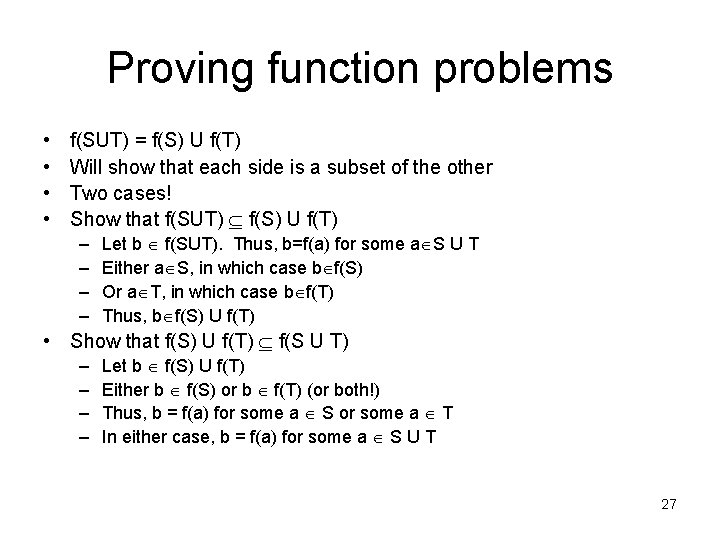Proving function problems • • f(SUT) = f(S) U f(T) Will show that each side is a subset of the other Two cases! Show that f(SUT) f(S) U f(T) – – Let b f(SUT). Thus, b=f(a) for some a S U T Either a S, in which case b f(S) Or a T, in which case b f(T) Thus, b f(S) U f(T) • Show that f(S) U f(T) f(S U T) – – Let b f(S) U f(T) Either b f(S) or b f(T) (or both!) Thus, b = f(a) for some a S or some a T In either case, b = f(a) for some a S U T 27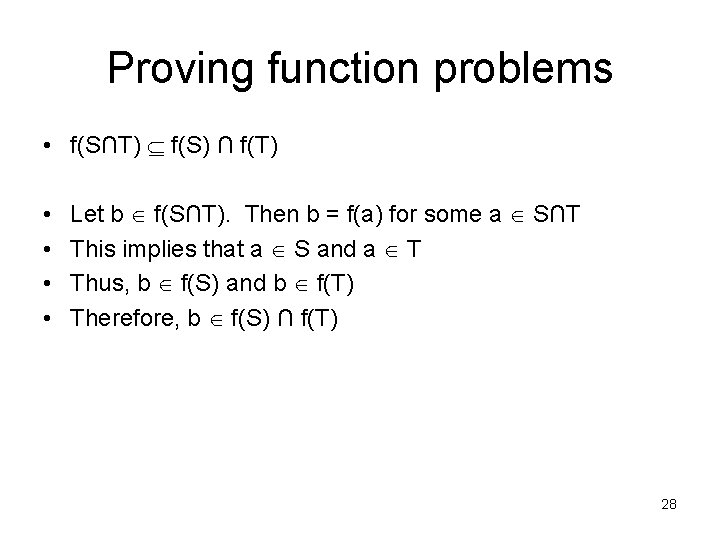Proving function problems • f(S∩T) f(S) ∩ f(T) • • Let b f(S∩T). Then b = f(a) for some a S∩T This implies that a S and a T Thus, b f(S) and b f(T) Therefore, b f(S) ∩ f(T) 28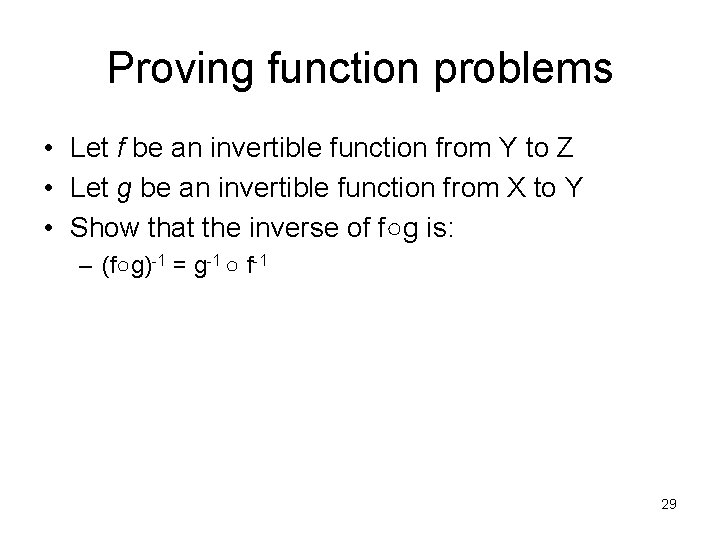Proving function problems • Let f be an invertible function from Y to Z • Let g be an invertible function from X to Y • Show that the inverse of f○g is: – (f○g)-1 = g-1 ○ f-1 29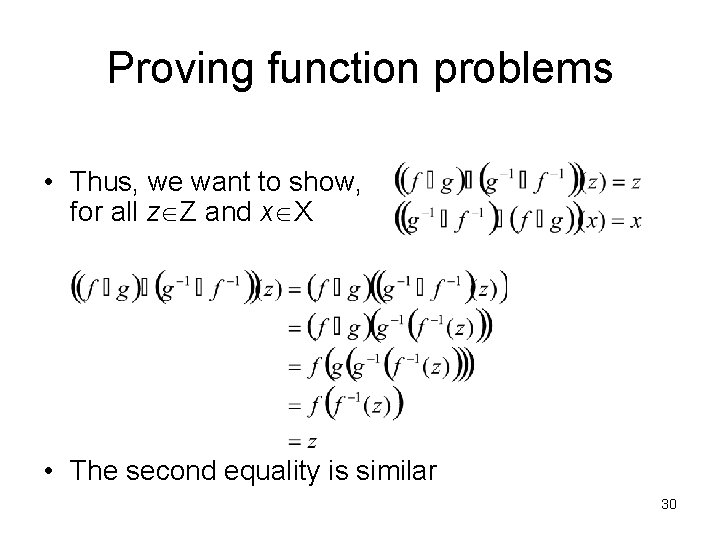Proving function problems • Thus, we want to show, for all z Z and x X • The second equality is similar 30